# A.4.16/17 Practice Problems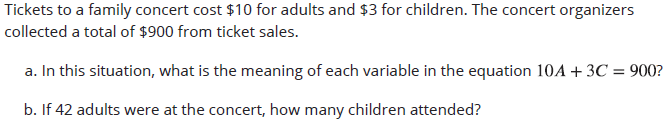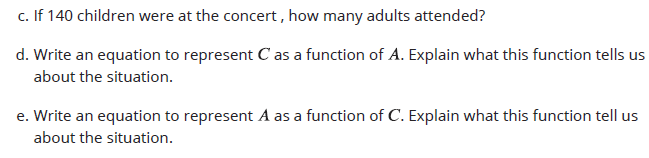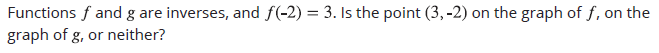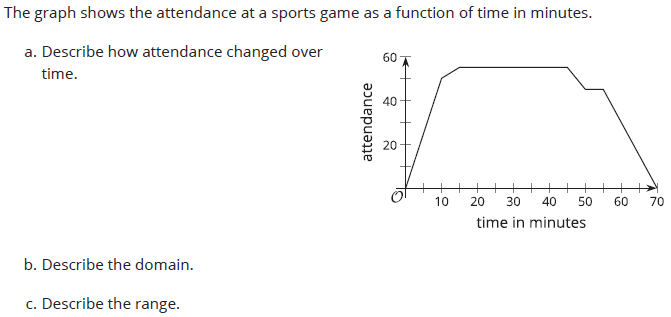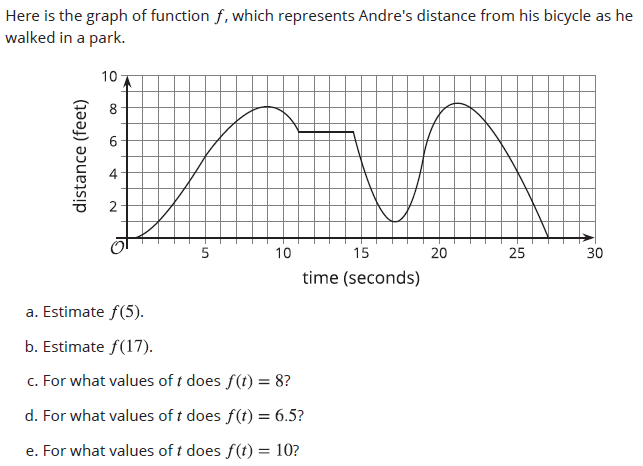Match each function to its inverse. A. y=2x-3 ﻿ ﻿1. x=(y+2)/3 B. y=3x ﻿ ﻿ ﻿ 2. x=(y+3)/2 C. y=3x-2 ﻿ ﻿ 3. x=3y+2 D. y=x-2 ﻿ ﻿4. x=y+2 E. y=x+2 ﻿ ﻿5. x=y/3 F. y=(x-2)/3 ﻿ 6. x=y-2

Crickets make chirping sounds by rubbing their wings together. The number of chirps they make is closely related to the temperature of their environment. When the temperature is between 55 and 100 degrees Fahrenheit, we can tell the temperature by counting the number of chirps!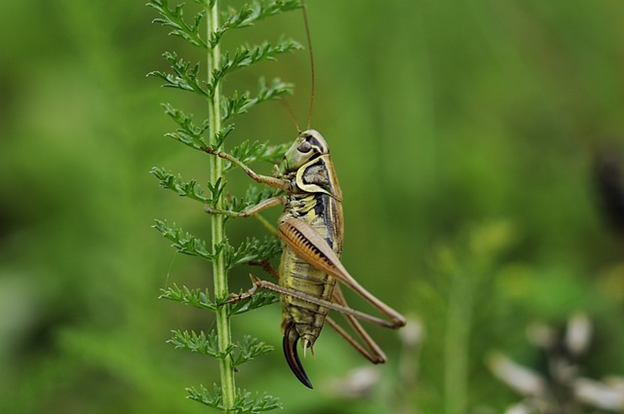A formula that is commonly used to find the temperature in degrees Fahrenheit is: Count the number of chirps in 14 seconds, and then add 40 to get the temperature. Let n be the number of chirps that crickets make in 14 seconds and F be the temperature in degrees Fahrenheit. a. What is the temperature when a cricket chirps 52 times in 14 seconds? b. Write an equation that defines F as a function of n. c. How many chirps would we expect to hear in 14 seconds when it is 60 degrees Fahrenheit? d. Write an equation that defines n as a function of F.